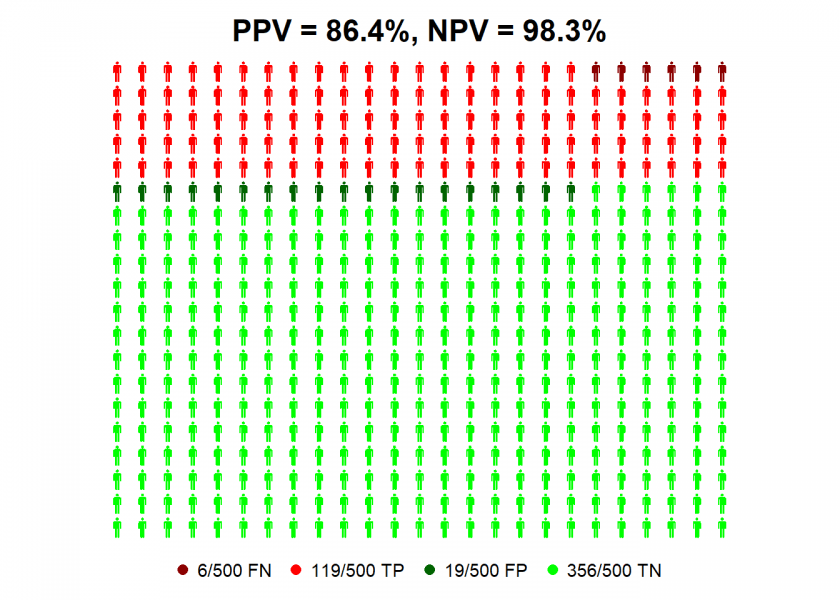Want to share your content on R-bloggers? click here if you have a blog, or here if you don't.In this post, we are going to replicate an analysis from the current issue of Scientific American about a common mathematical pitfall of Coronavirus antibody tests with R.

Many people think that when they get a positive result of such a test they are immune to the virus with high probability. If you want to find out why nothing could be further from the truth, read on!

The following article provides a good intuition of why the accuracy of screening tests is highly dependent on the infection rate: Scientific American: Coronavirus Antibody Tests Have a Mathematical Pitfall. They write:

A mathematical wrinkle makes these tests—and in fact, all screening tests—hard to interpret: even with a very accurate test, the fewer people in a population who have a condition, the more likely it is that an individual’s positive result is wrong. If it is, people might think they have the antibodies (and thus may have immunity), when in fact they do not.

We covered this concept in another post already (see Base Rate Fallacy – or why No One is justified to believe that Jesus rose) but because of the importance of the topic let us replicate their analysis with personograph plots (also called Kuiper-Marshall plots) which are an excellent way to communicate risks (see also Lying with Statistics: One Beer a Day will Kill you!).

Let us first repeat the definitions of sensitivity and specificity (we covered those here already: Learning Data Science: Understanding ROC Curves):

• Sensitivity or True Positive Rate (TPR): the proportion of actual (= true) positives (TP) that are correctly identified as such (e.g. the percentage of sick people who are correctly identified as having (had) the condition).
• Specificity or True Negative Rate (TNR): the proportion of actual (= true) negatives (TN) that are correctly identified as such (e.g. the percentage of healthy people who are correctly identified as not having (had) the condition).

That might be quite interesting but what really counts in the end aren’t any test results but whether you actually have (had) the virus or not! Those are two different things and therefore we define two additional measures:

• Positive predictive value (PPV): the probability that subjects with a positive screening test truly have (had) the disease.
• Negative predictive value (NPV): the probability that subjects with a negative screening test truly don’t have (have not had) the disease.

Please note that all four figures are conditional probabilities, but are going in different directions. For example, TPR gives the probability of a positive test result given you are sick, while PPV gives the probability of you being sick given a positive test result. We will see that both numbers can (and actually do!) diverge quite considerably.

Now we have everything in place to replicate the analysis with R (the `personograph` package can be found on CRAN). First with an infection rate of 5% (which is in the ballpark of the likely infection rate for most countries at the moment):

```library(personograph)
colorlist <- list(FN = "darkred", TP = "red", FP = "darkgreen", TN = "green")

TPR <- 0.95 # true positive rate = sensitivity
TNR <- 0.95 # true negative rate = specificity

IR <- 0.05 # infection rate
data <- list(FN = (1-TPR)*IR, TP = TPR*IR, FP = (1-TNR)*(1-IR), TN = TNR*(1-IR))
NPV <- round(100 * data\$TN / (data\$TN + data\$FN), 1) # negative predictive value
PPV <- round(100 * data\$TP / (data\$TP + data\$FP), 1) # positive predictive value
personograph(data, colors = colorlist, fig.title = paste0("PPV = ", PPV, "%, NPV = ", NPV, "%"), n.icons = 500, dimensions = c(20, 25))
```So we can see that even with antibody tests with high values for sensitivity and specificity a positive test result is not better than the toss of a coin, given the current infection rate! Why? Because for every true positive case (TP, in red) there is a false positive one (FP, in dark green).

The situation gets a better with an infection rate of 25%…

```IR <- 0.25 # infection rate
data <- list(FN = (1-TPR)*IR, TP = TPR*IR, FP = (1-TNR)*(1-IR), TN = TNR*(1-IR))
NPV <- round(100 * data\$TN / (data\$TN + data\$FN), 1) # negative predictive value
PPV <- round(100 * data\$TP / (data\$TP + data\$FP), 1) # positive predictive value
personograph(data, colors = colorlist, fig.title = paste0("PPV = ", PPV, "%, NPV = ", NPV, "%"), n.icons = 500, dimensions = c(20, 25))
```…where you can be nearly 90% confident that a positive test result means that you have (had) the virus! All of this shows the massive impact infection rates can have on the predictive value of these tests for individuals.

If you still doubt this I give you another intuition: the less probable it is that you have (had) the virus (which is the case right now because of the low infection rate) the more evidence you will need to prove the contrary, either by better tests (which we either don’t have at the moment or which are prioritized for the sickest patients) or by additional tests (which are scarcely done because of still limited resources).

On the other hand, if you get a negative result you can be pretty sure that you indeed have not had the virus!